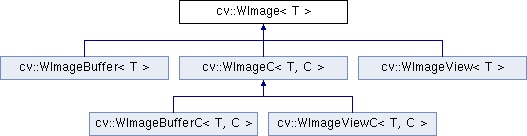OpenCV  3.0.0 Open Source Computer Vision
cv::WImage< T > Class Template Referenceabstract

Image class which provides a thin layer around an IplImage. More...

#include "wimage.hpp"

Inheritance diagram for cv::WImage< T >:## Public Types

typedef T BaseType

## Public Member Functions

virtual ~WImage ()=0

int Channels () const

int ChannelSize () const

void CopyFrom (const WImage< T > &src)

int Depth () const

template<>
int Depth () const

template<>
int Depth () const

template<>
int Depth () const

template<>
int Depth () const

template<>
int Depth () const

template<>
int Depth () const

template<>
int Depth () const

int Height () const

T * ImageData ()

const T * ImageData () const

IplImageIpl ()

const IplImageIpl () const

T * operator() (int c, int r)

const T * operator() (int c, int r) const

int PixelSize () const

const T * Row (int r) const

T * Row (int r)

void SetZero ()

WImageView< T > View (int c, int r, int width, int height)

int Width () const

int WidthStep () const

## Protected Member Functions

WImage (const WImage &)

WImage (IplImage *img)

void operator= (const WImage &)

void SetIpl (IplImage *image)

IplImageimage_

## Detailed Description

### template<typename T> class cv::WImage< T >

Image class which provides a thin layer around an IplImage.

The goals of the class design are:

-# All the data has explicit ownership to avoid memory leaks
-# No hidden allocations or copies for performance.
-# Easy access to OpenCV methods (which will access IPP if available)
-# Can easily treat external data as an image
-# Easy to create images which are subsets of other images
-# Fast pixel access which can take advantage of number of channels if known at compile time.


The WImage class is the image class which provides the data accessors. The 'W' comes from the fact that it is also a wrapper around the popular but inconvenient IplImage class. A WImage can be constructed either using a WImageBuffer class which allocates and frees the data, or using a WImageView class which constructs a subimage or a view into external data. The view class does no memory management. Each class actually has two versions, one when the number of channels is known at compile time and one when it isn't. Using the one with the number of channels specified can provide some compile time optimizations by using the fact that the number of channels is a constant.

We use the convention (c,r) to refer to column c and row r with (0,0) being the upper left corner. This is similar to standard Euclidean coordinates with the first coordinate varying in the horizontal direction and the second coordinate varying in the vertical direction. Thus (c,r) is usually in the domain [0, width) X [0, height)

Example usage:

WImageBuffer3_b im(5,7); // Make a 5X7 3 channel image of type uchar
WImageView3_b sub_im(im, 2,2, 3,3); // 3X3 submatrix
vector<float> vec(10, 3.0f);
WImageView1_f user_im(&vec, 2, 5); // 2X5 image w/ supplied data
im.SetZero(); // same as cvSetZero(im.Ipl())
*im(2, 3) = 15; // Modify the element at column 2, row 3
MySetRand(&sub_im);
// Copy the second row into the first. This can be done with no memory
// allocation and will use SSE if IPP is available.
int w = im.Width();
im.View(0,0, w,1).CopyFrom(im.View(0,1, w,1));
// Doesn't care about source of data since using WImage
void MySetRand(WImage_b* im) { // Works with any number of channels
for (int r = 0; r < im->Height(); ++r) {
float* row = im->Row(r);
for (int c = 0; c < im->Width(); ++c) {
for (int ch = 0; ch < im->Channels(); ++ch, ++row) {
*row = uchar(rand() & 255);
}
}
}
}

Functions that are not part of the basic image allocation, viewing, and access should come from OpenCV, except some useful functions that are not part of OpenCV can be found in wimage_util.h

## Member Typedef Documentation

template<typename T>
 typedef T cv::WImage< T >::BaseType

## Constructor & Destructor Documentation

template<typename T>
 cv::WImage< T >::WImage ( const WImage< T > & )
protected
template<typename T>
 cv::WImage< T >::WImage ( IplImage * img )
inlineexplicitprotected

## Member Function Documentation

template<typename T>
 int cv::WImage< T >::Channels ( ) const
inline
template<typename T>
 int cv::WImage< T >::ChannelSize ( ) const
inline
template<typename T>
 void cv::WImage< T >::CopyFrom ( const WImage< T > & src )
inline
template<typename T>
 int cv::WImage< T >::Depth ( ) const
template<>
 int cv::WImage< uchar >::Depth ( ) const
inline
template<>
 int cv::WImage< signed char >::Depth ( ) const
inline
template<>
 int cv::WImage< short >::Depth ( ) const
inline
template<>
 int cv::WImage< ushort >::Depth ( ) const
inline
template<>
 int cv::WImage< int >::Depth ( ) const
inline
template<>
 int cv::WImage< float >::Depth ( ) const
inline
template<>
 int cv::WImage< double >::Depth ( ) const
inline
template<typename T>
 int cv::WImage< T >::Height ( ) const
inline
template<typename T>
 T* cv::WImage< T >::ImageData ( )
inline
template<typename T>
 const T* cv::WImage< T >::ImageData ( ) const
inline
template<typename T>
 IplImage* cv::WImage< T >::Ipl ( )
inline
template<typename T>
 const IplImage* cv::WImage< T >::Ipl ( ) const
inline
template<typename T>
 T* cv::WImage< T >::operator() ( int c, int r )
inline
template<typename T>
 const T* cv::WImage< T >::operator() ( int c, int r ) const
inline
template<typename T>
 void cv::WImage< T >::operator= ( const WImage< T > & )
protected
template<typename T>
 int cv::WImage< T >::PixelSize ( ) const
inline
template<typename T>
 const T* cv::WImage< T >::Row ( int r ) const
inline
template<typename T>
 T* cv::WImage< T >::Row ( int r )
inline
template<typename T>
 void cv::WImage< T >::SetIpl ( IplImage * image )
inlineprotected
template<typename T>
 void cv::WImage< T >::SetZero ( )
inline
template<typename T>
 int cv::WImage< T >::Width ( ) const
inline
template<typename T>
 int cv::WImage< T >::WidthStep ( ) const
inline

## Member Data Documentation

template<typename T>
 IplImage* cv::WImage< T >::image_
protected

The documentation for this class was generated from the following file: